Technical Article

# The Nyquist–Shannon Theorem in the Frequency Domain

May 07, 2020 by Robert Keim

## This article continues our discussion of sampling theory and introduces the concept of aliasing.

In the previous article introducing the Nyquist-Shannon theorem, we saw that the frequency characteristics of a sinusoid are irretrievably lost when the waveform is sampled at a frequency that does not provide at least two samples per cycle. In other words, we cannot perfectly reconstruct the sinusoid if we sample at a frequency that is lower than the Nyquist rate.

Most signals, however, are not single-frequency sinusoids. For example, a modulated RF signal has frequencies associated with the carrier and the baseband waveform, and an audio signal representing human speech will cover a range of frequencies.

We use the Fourier transform to visualize the frequency content of a signal. Time-domain plots are a good way to convey the effect of insufficient sampling rate in the context of a single-frequency signal, but for other types of signals, I’d rather use the frequency domain.

### Frequency-Domain Effect of Sampling

Let’s say that we want to digitize an audio signal that includes a mixture of many different frequencies within a specified range. The high end of the range is defined as fMAX, and we’ll assume that the range extends down to DC, even though we can’t hear frequencies that low. The Fourier transform of such a signal might look something like this: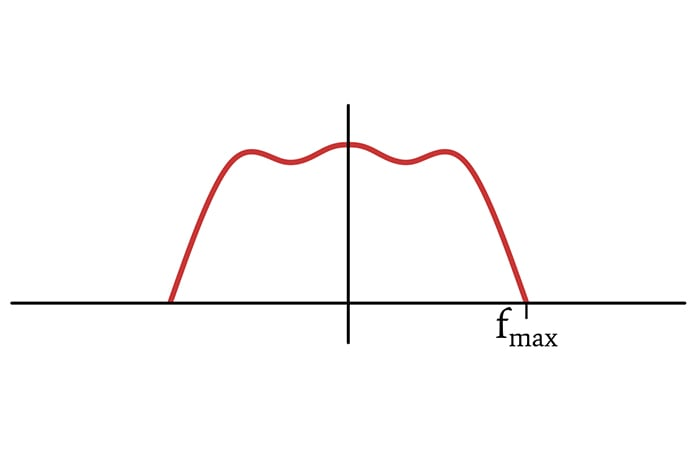#### Mathematical Sampling in the Time Domain

In the mathematical realm, ideal sampling is equivalent to multiplying the original time-domain waveform by a train of delta functions separated by an interval equal to 1/fSAMPLE, which we’ll call TSAMPLE. (For the rest of the article, we’ll use fS for fSAMPLE and TS for TSAMPLE.) This multiplication causes the sampled signal to be zero between the delta functions and to retain the value of the original signal at each point in time that coincides with a delta function.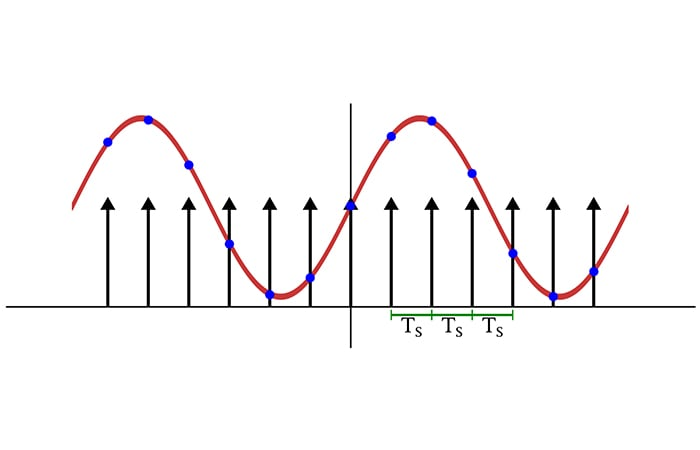#### Mathematical Sampling in the Frequency Domain

How does this time-domain sampling procedure affect the frequency-domain representation of a signal? Let’s take a look.

The first thing to remember is that multiplication in the time domain becomes convolution in the frequency domain. Thus, we can find the Fourier transform of the sampled signal by convolving the Fourier transform of the original signal with the Fourier transform of the delta functions.

It turns out that the Fourier transform of a delta-function train is a delta-function train. The difference is that the delta functions are separated by a horizontal distance corresponding to the sampling frequency rather than the sampling period.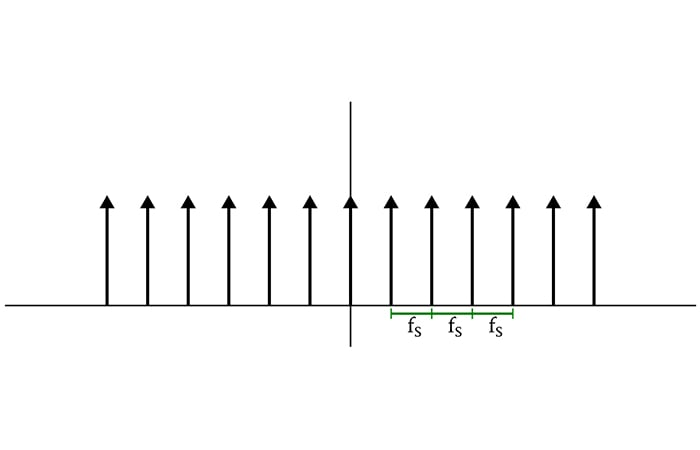##### The spectrum of a sequence of delta functions separated by the sampling period is a sequence of delta functions separated by the sampling frequency.

When we convolve the spectrum of the delta functions with the spectrum of the original signal, we create copies of the original spectrum that are shifted according to the position of the delta functions. Thus, the spectrum of a sampled signal consists of multiple identical “subspectra” that are centered on ±fS, ±2fS, ±3fS, and so on.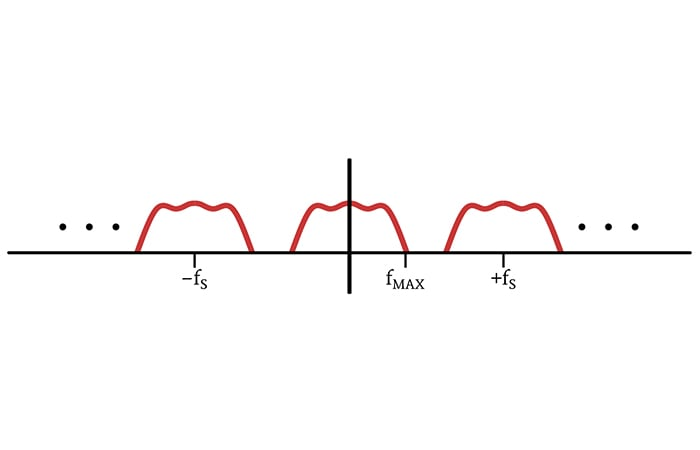##### Adequate sampling frequency results in subspectra that are shifted enough to maintain full separation.

We now have the information we need to confirm the Nyquist–Shannon theorem via frequency-domain analysis. This theorem, as I expressed it in the previous article, is the following:

If a system uniformly samples an analog signal at a rate that exceeds the signal’s highest frequency by at least a factor of two, the original analog signal can be perfectly recovered from the discrete values produced by sampling.

Because of the negative-frequency portion of the Fourier transform, the full mathematical bandwidth of the original signal is 2fMAX. Thus, in order to ensure that the subspectra do not overlap, we must shift them by at least 2fMAX. In other words, the sampling frequency must be higher than the signal’s maximum frequency by at least a factor of two.

If this condition is met, the original signal can be perfectly reconstructed. Why? Because the original spectrum has not been altered, and we can eliminate the other subspectra via low-pass filtering. (The next article will explore this point in more detail.) If the condition is not met, the subspectra overlap, the original spectrum is altered, and no amount of low-pass filtering will restore the original signal.

### Aliasing

Subspectra overlap is the reason why information is corrupted when we use a sampling frequency that is lower than the Nyquist rate. The overlapping sections of subspectra combine through addition; if we try to separate out the original spectrum using a low-pass filter, the frequency content in the overlapping bands will be different, and consequently the corresponding time-domain signal will be different.

The official name for this is aliasing.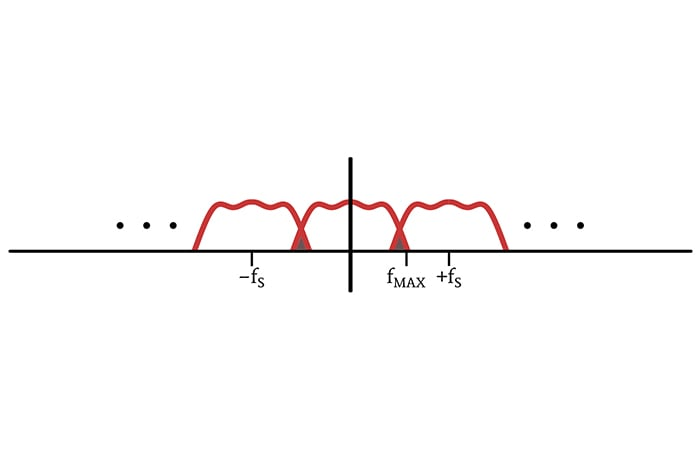##### The triangular areas shaded in brown represent aliasing that has caused spectral alteration.

One of the definitions of the noun “alias” is “a false or assumed identity.” We use the term “aliasing” because this sampling phenomenon can cause one frequency component to shift into a new location in the spectrum and thereby “disguise” itself as a different frequency.

We saw this in the previous article, where sampling at 1.1fSIGNAL resulted in a discrete-time waveform that appeared to have a frequency much lower than the frequency of the original analog waveform.

### Conclusion

At this point, I think that we’ve covered the foundational aspects of sampling theory. In the next article, we’ll start to form some connections between theory and practice.• B
Bernie Hutchins May 14, 2020

In the “Fourier Transform” blurb referenced here there is a fundamental misunderstanding!  It shows a theoretical case of the superposition of a 1 MHz sine with a 2 MHz sine (“IDEAL” line spectra) along with a corresponding case of a practical (“REAL”) superposition where the two components are broadened lobes.  The broadening is blamed on limited resolution and/or noise, while the real cause is the FINITE DURATION (so-called “windowing”) of the real signal.  This is a serious misunderstanding!

Only infinite duration sinusoids can have zero bandwidth. Once you make the durations finite (all practical signals) you have the signal multiplied (in time) by a window.  The multiplication becomes convolution in the frequency domain.  Effectively the FT of the window is copied about the lines.  This is the broadening seen in the real case.  [If the window use for the plot were rectangular (simple truncation) the lobes would be sinc functions.  The sidelobes here are so small here, something like a Hamming windrow appears more likely (author?)]

“Resolution” (effectively the width of the main lobe of the window’s FT, improves with the length off the signal (more cycles considered – “uncertainty relation”) but this result is due to the same fundamental cause (windowing), and noise has nothing to do with it.

Like.
•RK37 May 15, 2020
Thanks for the comment. I see where you're coming from, but noise/frequency variation would indeed cause the real spectrum to deviate (in some way) from the idealized mathematical spectrum, and this is the primary point that the other article is trying to make. And it seems to me that phase noise does contribute to the broadened spectrum shape shown in the diagram. I find other examples online of this spectrum shape used to demonstrate the effect of phase noise on an idealized sinusoid. For example: https://www.researchgate.net/figure/Schematic-illustration-of-a-the-ideal-sinusoidal-signal-and-its-power-spectrum-density_fig3_334104041 https://synergymwave.com/articles/2007/09/images/AR_4866_Fig02_L.jpg
Like.
• B
Bernie Hutchins May 15, 2020
Robert - Thanks. I am guessing that you are the author of the “Fourier transform” reference (?). If so, or if you know, exactly how was the FT setup and calculated? Is it a Fourier integral of a windowed segment? Is it an FFT? Was any noise (additive; or frequency or phases shift) even added? Somehow it is broadened. How? Is not my explanation (just a basic a windowing result) almost certainly wholly sufficient, and correct? - Bernie
Like.